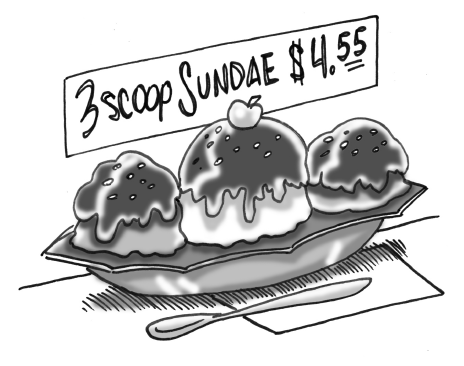### Home > INT1 > Chapter 11 > Lesson 11.2.1 > Problem11-54

11-54.

Pierre’s Ice Cream Shoppe charges $1.19$ for a scoop of ice cream and $0.49$ for each topping. Gordon paid $4.55$ for a three-scoop sundae. How many toppings did he get?

Let $x$ represent the number of toppings Gordon ordered.

Create an equation relating the $3$ scoops, the $x$ toppings, and the $4.55$ total.

$(3)(1.19) + (0.49)x = 4.55$